2021-05-17 204 4

# 解题思路

## 1. 暴力求解法1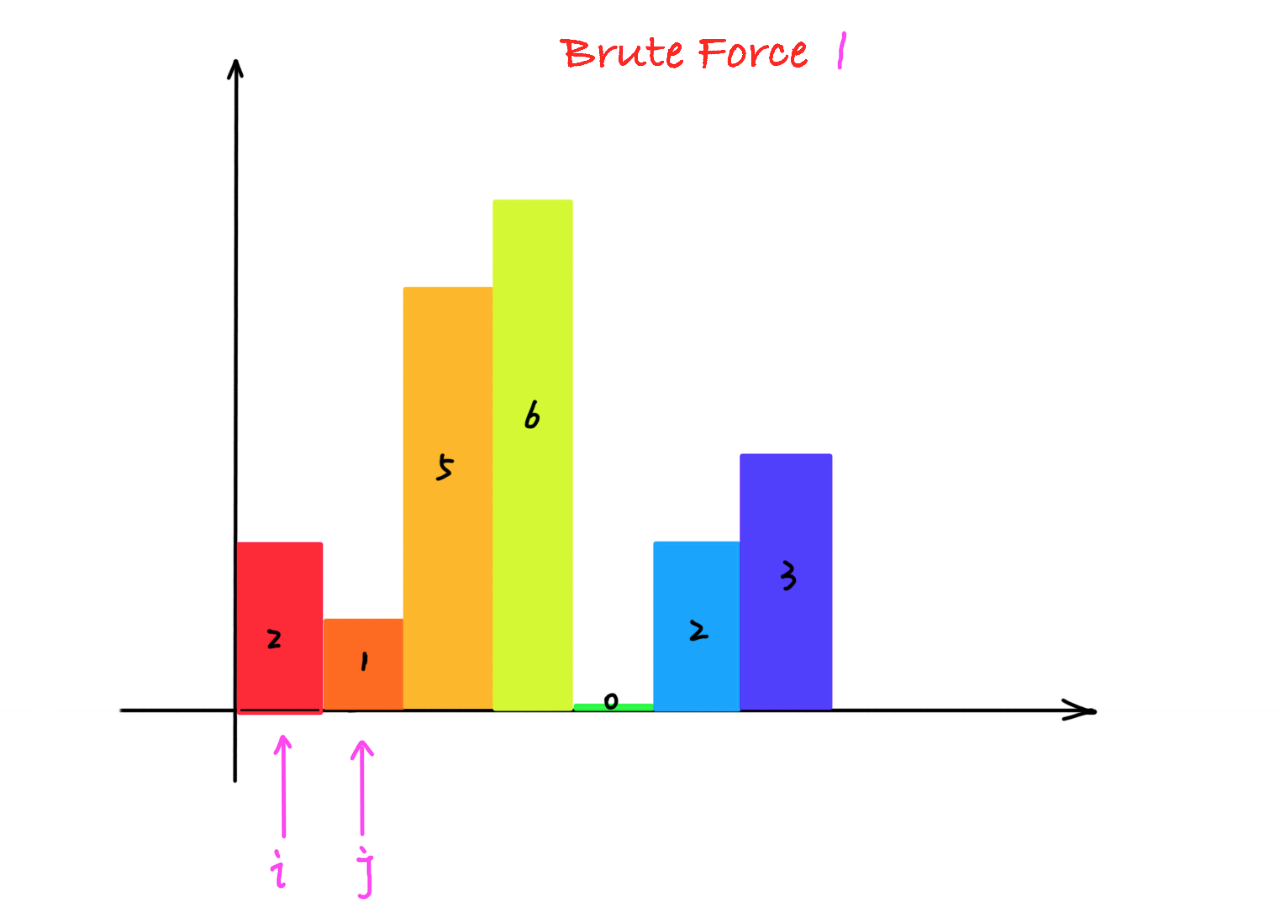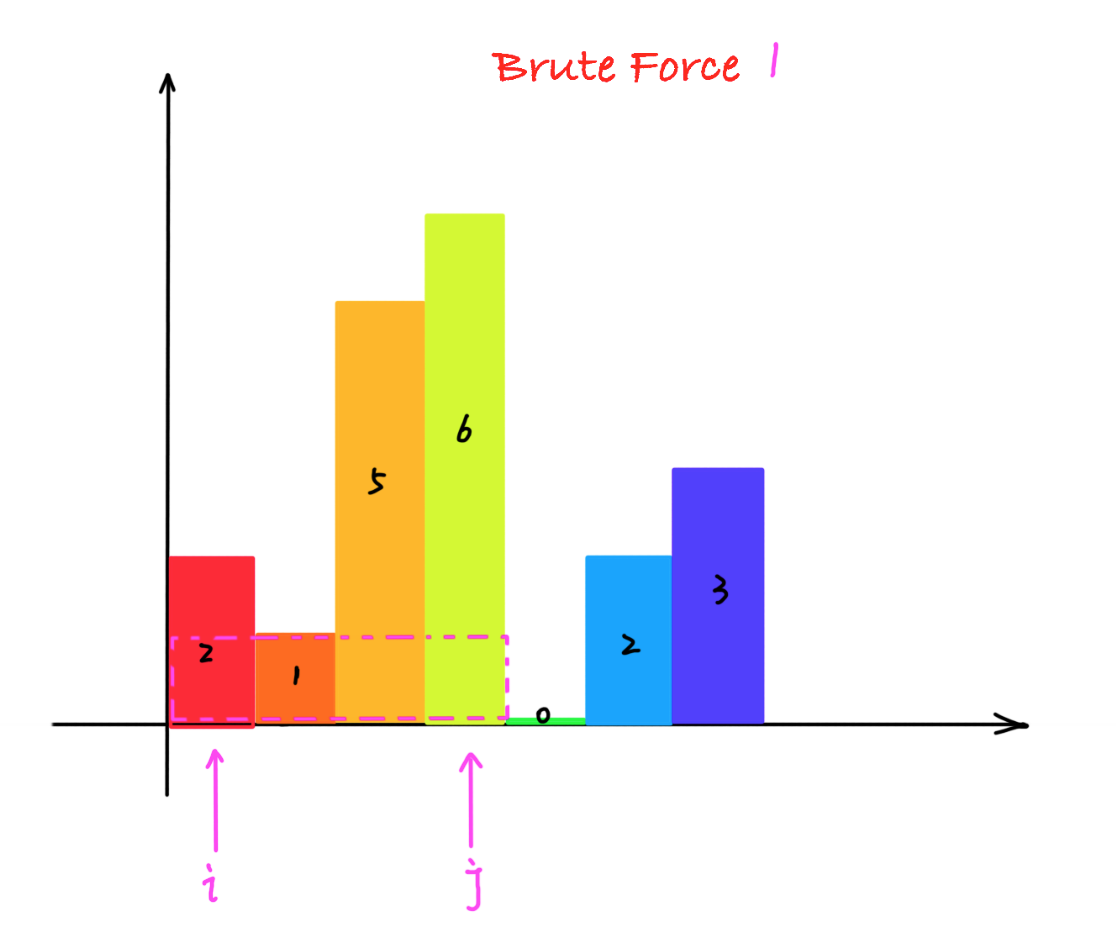`class` `Solution `
`{`
`public``:`
`    ``int` `largestRectangleArea(vector<``int``>& heights) `
`    ``{`
`        ``int` `length = heights.size();`
`        ``int` `minHeight;`
`        ``int` `maxArea = 0;`
`        ``int` `width = 1;`
`        ``for``(``int` `i = 0; i < length; ++i)`
`        ``{`
`            ``// 单个柱子的面积`

`            ``maxArea = maxArea >  heights[i] * 1 ? maxArea : heights[i] * 1;``   `

` `

`            ``// 重置 高和宽`
`            ``minHeight = heights[i];`

`            ``width = 1;`

`            ``// 组合柱子的面积`
`            ``for``(``int` `j = i + 1; j < length; ++j)`
`            ``{`
`                ``minHeight = minHeight < heights[j] ? minHeight : heights[j];`
`                ``++width;`
`                ``maxArea = maxArea > minHeight * width ? maxArea : minHeight * width;`
`            ``}`
`        ``} `
`        ``return` `maxArea;      `
`    ``}`
`};`

2. 暴力求解法2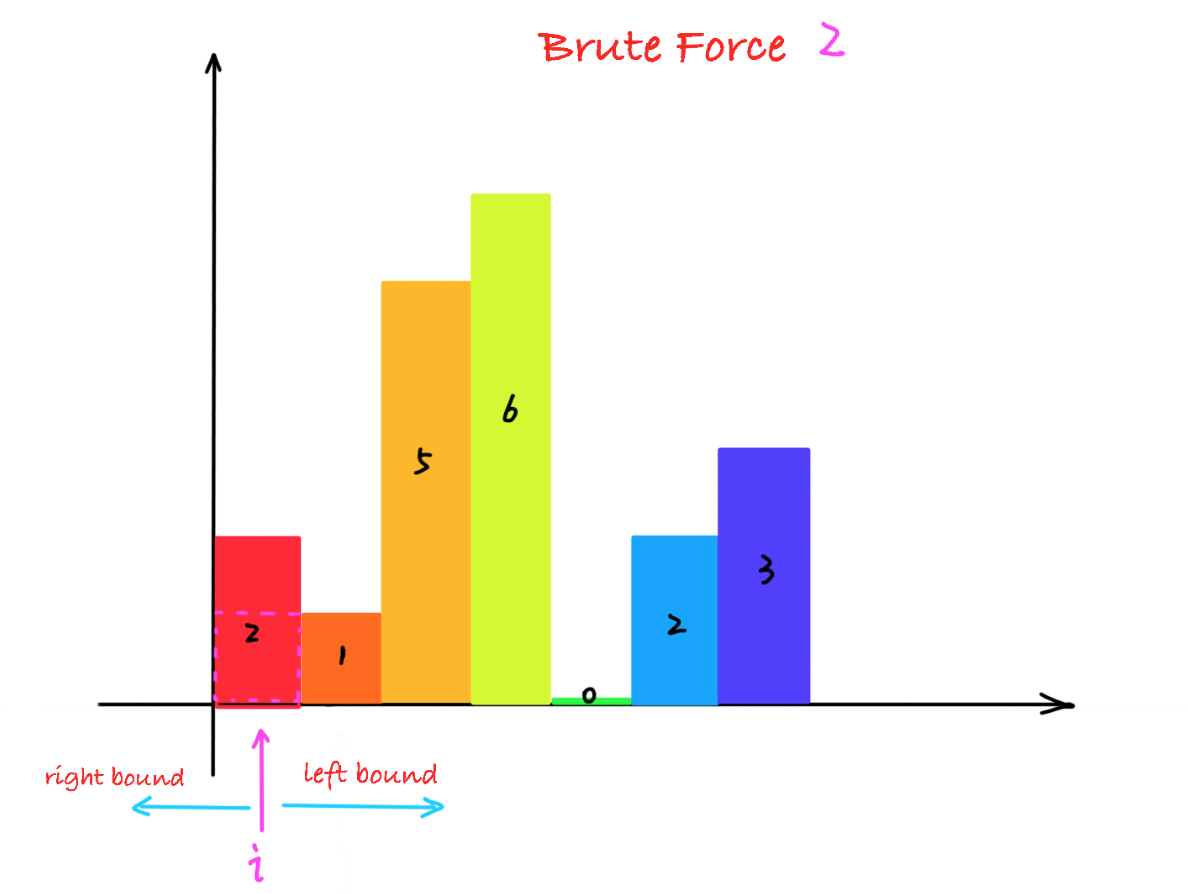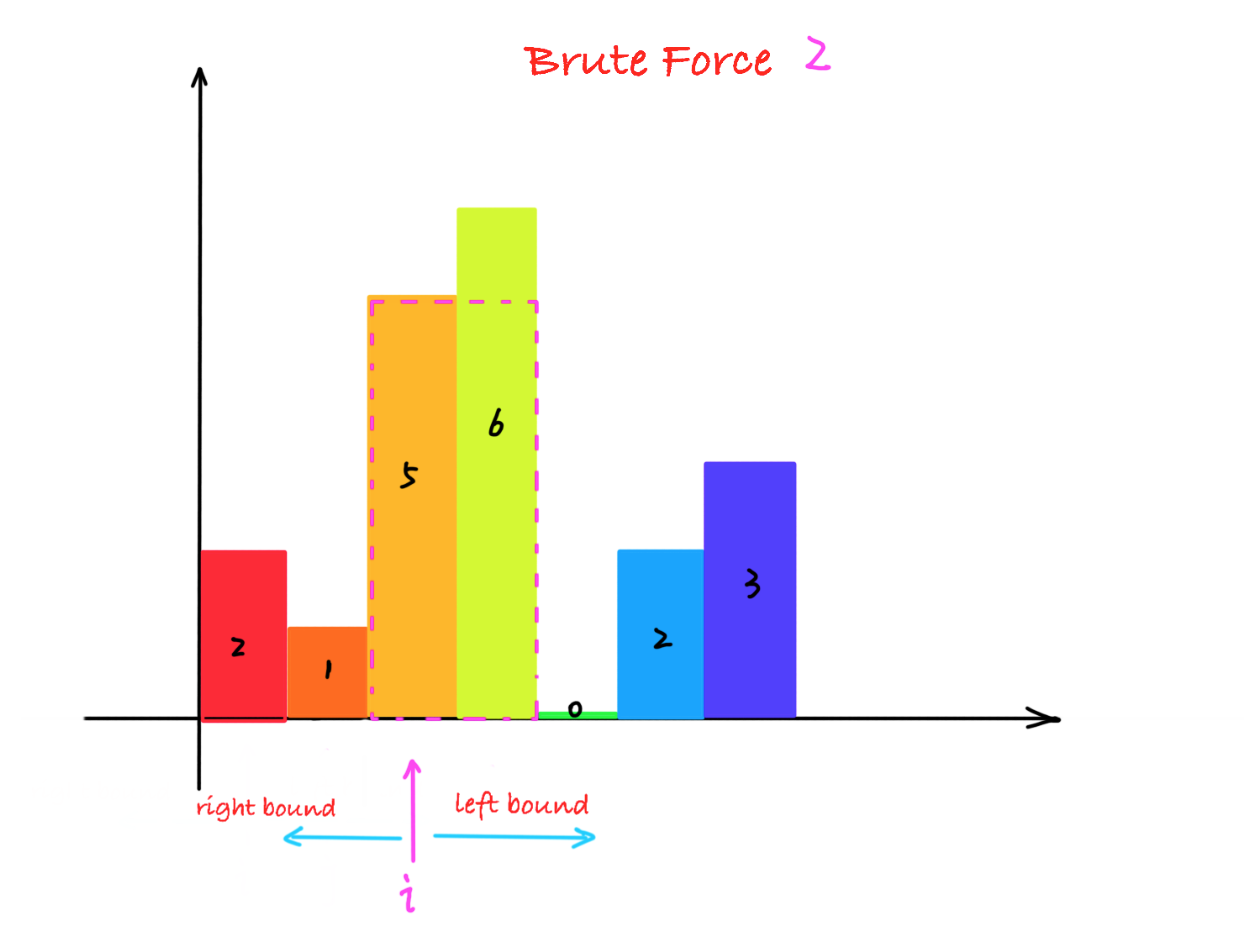`class` `Solution {`
`    ``public` `int` `largestRectangleArea(``int``[] heights) {`
`        ``int` `len = heights.length;`

`        ``int` `maxarea = ``0``;`

`        ``// 枚举每个柱子`
`        ``for``(``int` `i = ``0``; i < len; i++) {`
`            ``// 寻找左边界`
`            ``int` `left = ``0``;`
`            ``for``(left = i - ``1``; left >= ``0``; left--) {`
`                ``if``(heights[left] < heights[i])`
`                    ``break``;`

`            ``}`

`            ``int` `right = ``0``;`
`            ``// 寻找右边界`
`            ``for``(right = i + ``1``; right < len; right++) {`
`                ``if``(heights[right] < heights[i])`
`                    ``break``;`

`            ``}`

`            ``// 计算最大面积`
`            ``maxarea = Math.max(maxarea, heights[i] * (right - left - ``1``));`
`        ``}`
`        ``return` `maxarea;`
`    ``}`
`}`

## 3. 最终优化方案----递增栈

对于暴力求解法2，我们发现对于左右边界的查找是有规律的

1.如果柱子的高度递减，那么每个柱子的左边界就是第一根柱子，右边界就是本身。

2.如果柱子的高度递增，那么每个柱子的右边界就是最后一根柱子，左边界就是本身。

有了这样的思路，那么对于无序的柱子组合，我们可以将其拆分， 保证每一个局部是有序的，然后计算最大面积就容易了。

局部的柱子有序后，那如何保存这些局部的柱子呢？ 我们发现这些柱子，具有最近相关性，所以使用栈保存这些有序的柱子。

1. 遍历每一个柱子，如果当前遍历的柱子比栈顶大，那么当前柱子入栈，说明还没有找到右边界。

2. 如果当前遍历的柱子比栈顶小，那么栈顶的柱子找到了右边界，当前遍历柱子的左边界就是栈中栈顶元素的下一个元素的位置，这时候面积就可以计算了。

简单说来就是： 大则入栈，小则处理栈中元素计算最大面积，直到栈中没有比当前遍历的柱子更短的柱子，然后将当前遍历的柱子再入栈。

遍历结束后， 栈中可能有数据， 这时候的数据必然是递增的， 同时没根柱子的右边界是柱状图的最后一根柱子，每个柱子的左边界就是他本身，那么面积就可以很容易计算了。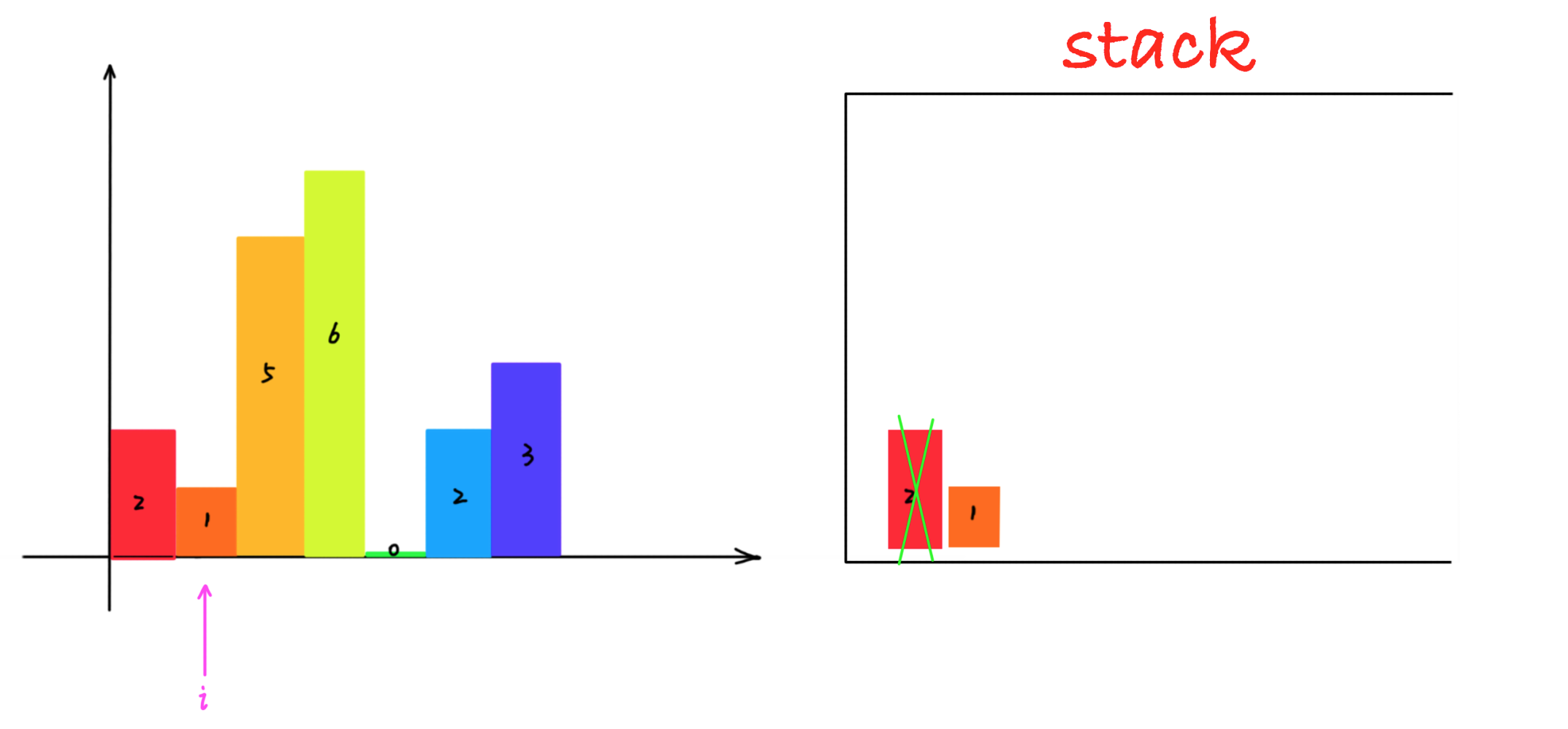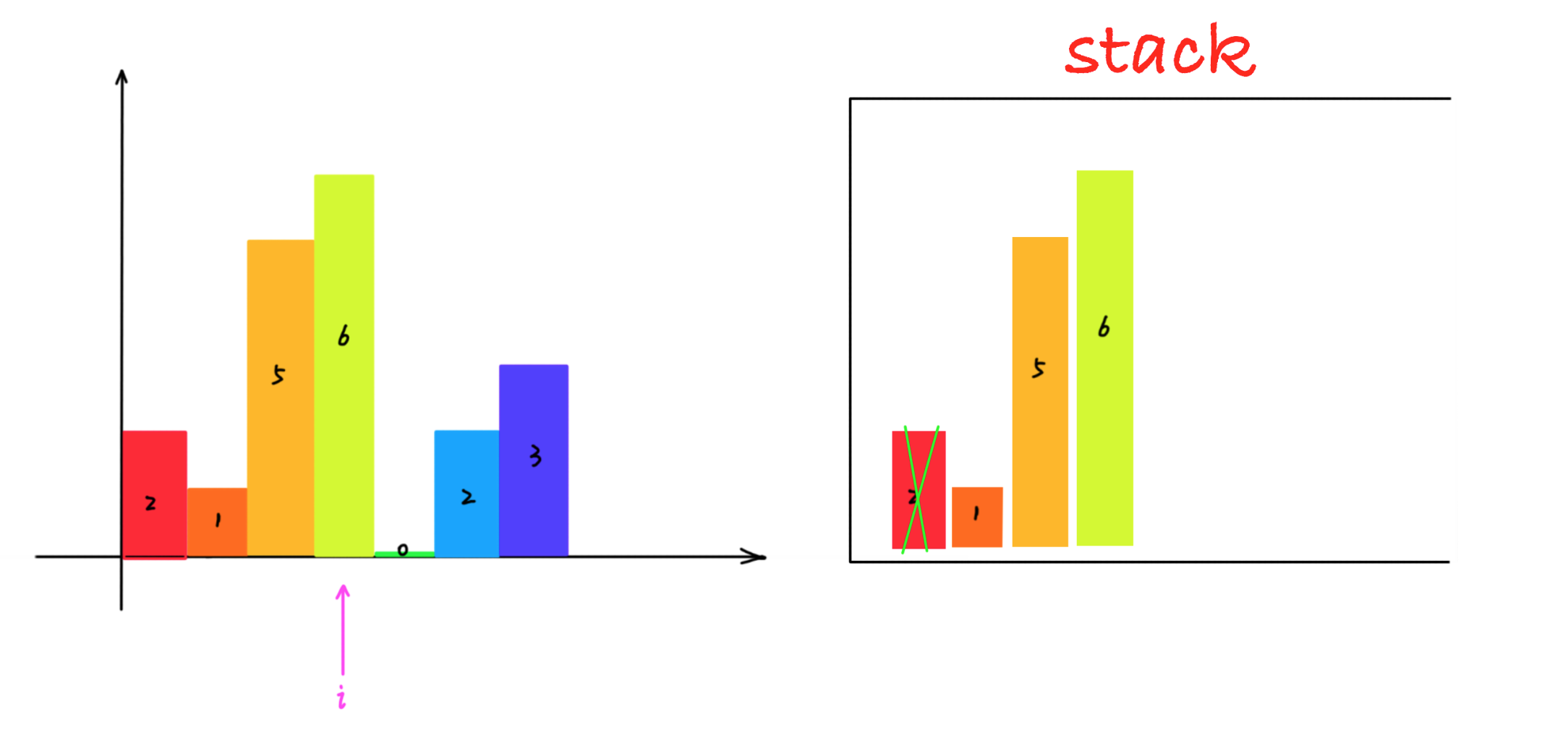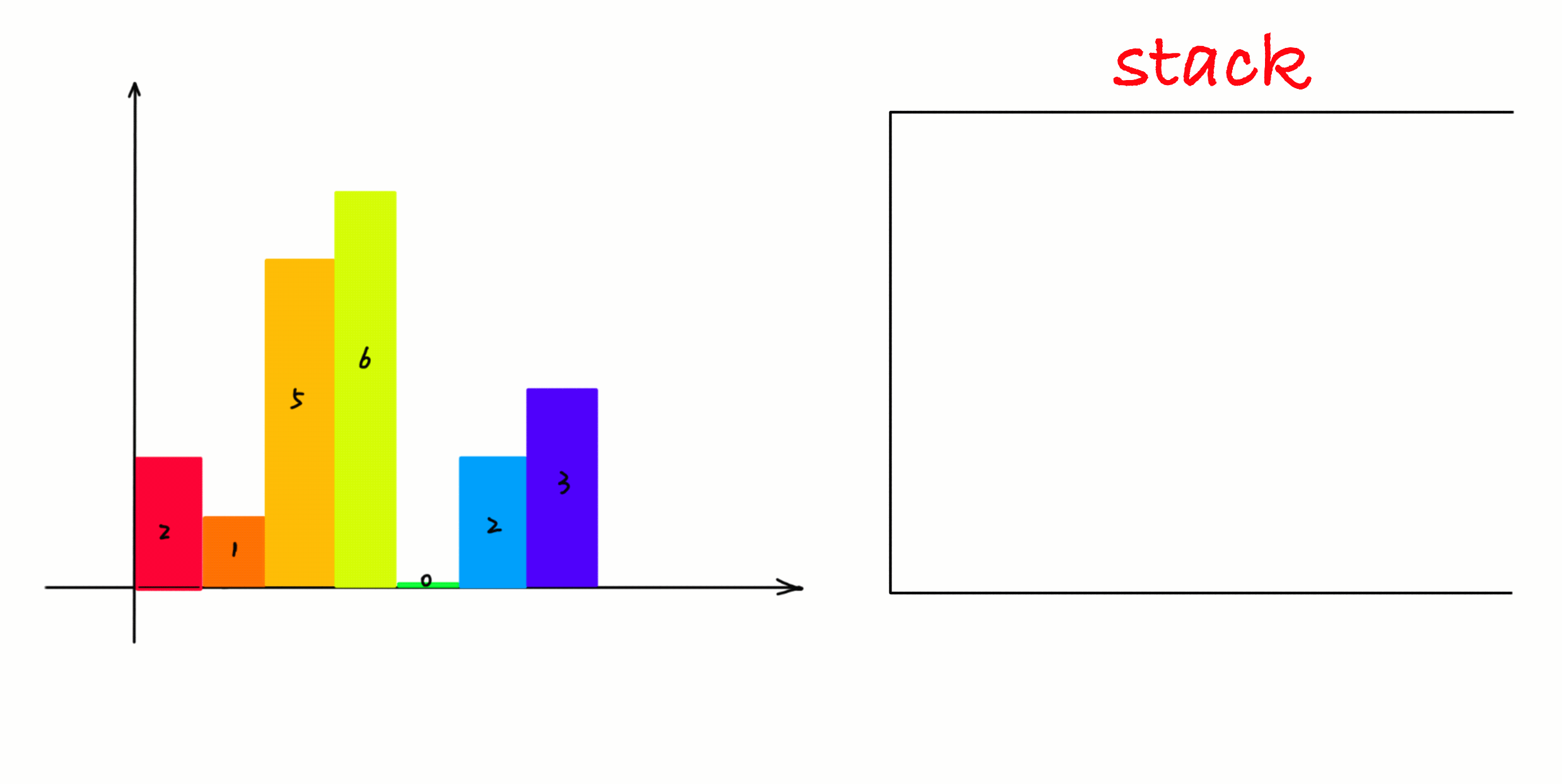`// C++`
`class` `Solution {`
`public``:`
`    ``int` `largestRectangleArea(vector<``int``>& heights) {`
`        ``int` `len = heights.size();`
`        ``int` `maxarea = 0;`

`        ``stack<``int``> stk;  `

`        ``for``(``int` `i = 0; i < len; ++i) {`
`            ``// 只要栈不为空，并且当前遍历的数据小于栈顶元素，则说明找到了右边界`
`            ``while``(!stk.empty() && heights[i] < heights[stk.top()]) {`
`                ``// 右边界 heights[i]`
`                ``int` `h = heights[stk.top()];`
`                ``stk.pop();`
`                ``// 出栈后，如果栈为空，说明出栈的柱子目前遍历的最短的柱子，否则计算宽度差`
`                ``int` `w = stk.empty() ? i : i - stk.top() - 1;`
`                ``maxarea = max(h * w, maxarea);`

`            ``}`

`            ``// 栈为空或者当前遍历的数据大于等于栈顶数据，则入栈，不会执行上面的while循环`
`            ``// 保证了栈中的数据总是递增的 比如  0 1 2 2 3 4 4 5 6 ...`
`            ``stk.push(i);`

`        ``}`

`        ``// 处理剩余栈中的数据(递增的数据，右边界是柱状图中最右边的柱子)`
`        ``while``(!stk.empty()) {`
`            ``int` `h = heights[stk.top()];`

`            ``stk.pop();`

`            ``// 出栈后，如果栈为空，说明出栈的柱子目前遍历的最短的柱子，否则计算宽度差`
`            ``int` `w = stk.empty() ? len : len - stk.top() - 1;`
`            ``maxarea = max(h * w, maxarea);`
`        ``}`
`}`

`// Java`
`class` `Solution {`
`    ``public` `int` `largestRectangleArea(``int``[] heights) {`
`        ``int` `len = heights.length;`
`        ``int` `maxarea = ``0``;`

`        ``Stack<Integer> stk = ``new` `Stack<Integer>();`

`        ``for``(``int` `i = ``0``; i < len; ++i) {`

`            ``// 只要栈不为空，并且当前遍历的数据小于栈顶元素，则说明找到了右边界`
`            ``while``(!stk.empty() && heights[i] < heights[stk.peek()]) {`
`                ``// 右边界 heights[i]`
`                ``int` `h = heights[stk.peek()];`
`                ``stk.pop();`
`                ``// 出栈后，如果栈为空，说明出栈的柱子目前遍历的最短的柱子，否则计算宽度差`
`                ``int` `w = stk.empty() ? i : i - stk.peek() - ``1``;`
`                ``maxarea = Math.max(h * w, maxarea);`

`            ``}`

`            ``// 栈为空或者当前遍历的数据大于等于栈顶数据，则入栈，不会执行上面的while循环`
`            ``// 保证了栈中的数据总是递增的 比如  0 1 2 2 3 4 4 5 6 ...`
`            ``stk.push(i);`

`        ``}`

`        ``// 处理剩余栈中的数据(递增的数据，右边界是柱状图中最右边的柱子)`
`        ``while``(!stk.empty()) {`
`            ``int` `h = heights[stk.peek()];`

`            ``stk.pop();`

`            ``// 出栈后，如果栈为空，说明出栈的柱子目前遍历的最短的柱子，否则计算宽度差`
`            ``int` `w = stk.empty() ? len : len - stk.peek() - ``1``;`
`            ``maxarea = Math.max(h * w, maxarea);`
`        ``}`
`        ``return` `maxarea;`
`    ``}`
`}`

`##Python`
`class` `Solution:`
`    ``def` `largestRectangleArea(``self``, heights: ``List``[``int``]) ``-``> ``int``:`

`        ``maxarea ``=` `0`
`        ``stk ``=` `list``()`

`        ``for` `i ``in` `range``(``len``(heights)):`
`            ``# 只要栈不为空，并且当前遍历的数据小于栈顶元素，则说明找到了右边界`
`            ``while` `stk ``and` `heights[i] < heights[stk[``-``1``]]:`
`                ``h ``=` `heights[stk[``-``1``]];`

`                ``stk.pop()`

`                ``# 出栈后，如果栈为空，说明出栈的柱子目前遍历的最短的柱子，否则计算宽度差`
`                ``w ``=` `i ``-` `stk[``-``1``] ``-` `1` `if` `stk ``else` `i`

`                ``maxarea ``=` `max``(h ``*` `w, maxarea)`

`            ``# 栈为空或者当前遍历的数据大于等于栈顶数据，则入栈，不会执行上面的while循环`
`            ``# 保证了栈中的数据总是递增的 比如  0 1 2 2 3 4 4 5 6 ...`
`            ``stk.append(i)`

`        ``# 处理剩余栈中的数据(递增的数据，右边界是柱状图中最右边的柱子)`
`        ``while` `stk:`
`            ``h ``=` `heights[stk[``-``1``]]`

`            ``stk.pop()`

`            ``# 出栈后，如果栈为空，说明出栈的柱子目前遍历的最短的柱子，否则计算宽度差`
`            ``w ``=` `len``(heights) ``-` `stk[``-``1``] ``-` `1` `if` `stk ``else` `len``(heights)`
`            ``maxarea ``=` `max``(h ``*` `w, maxarea)`
`        `
`        ``return` `maxarea`

## 文章评论112 文章 41435 浏览 3 评论